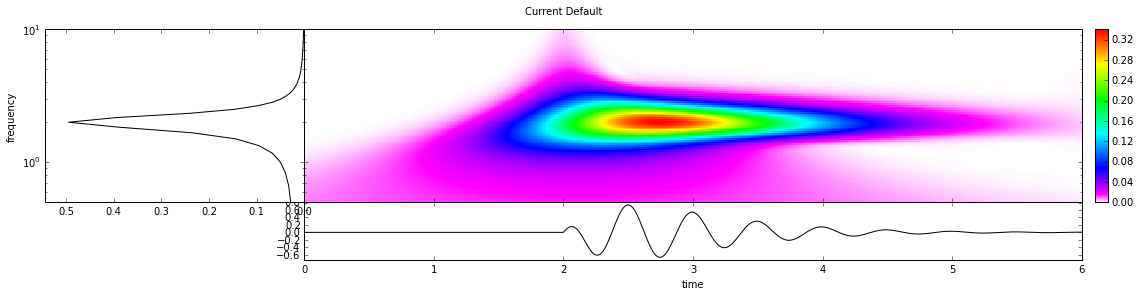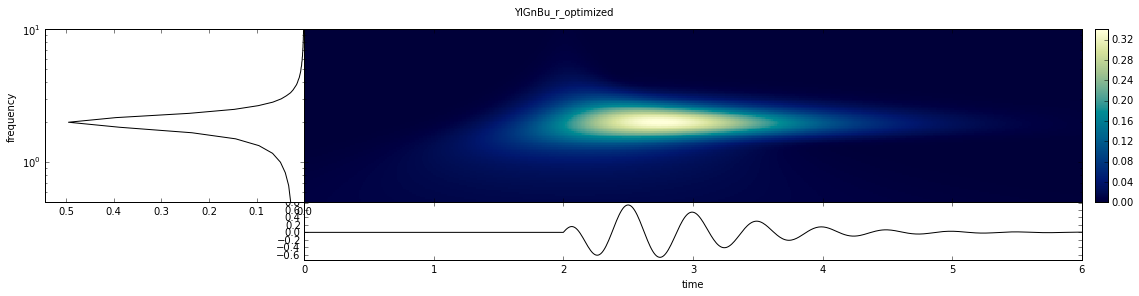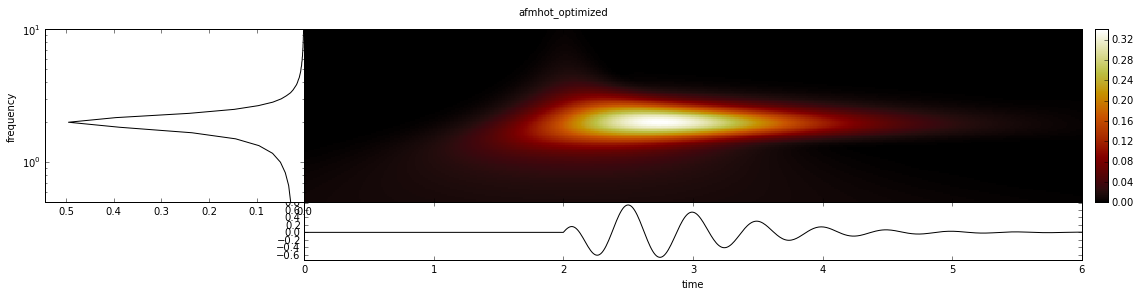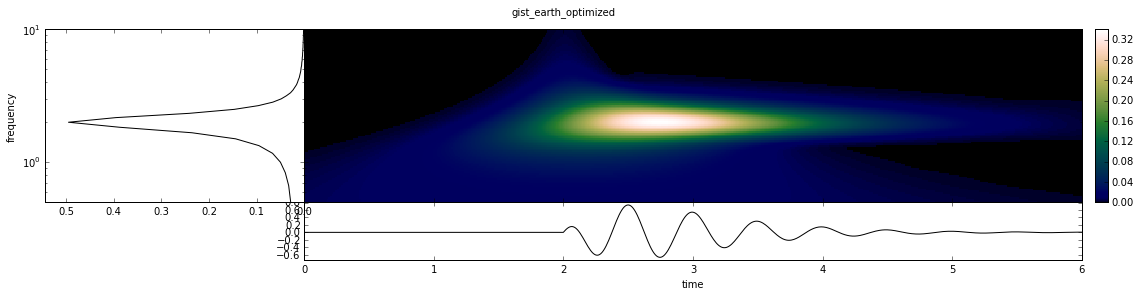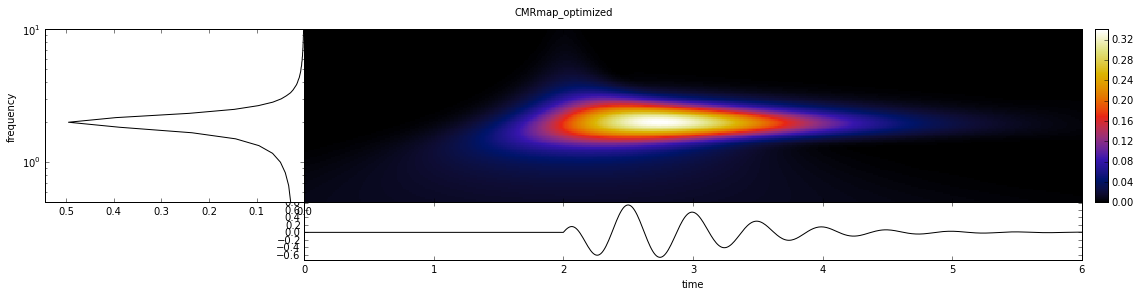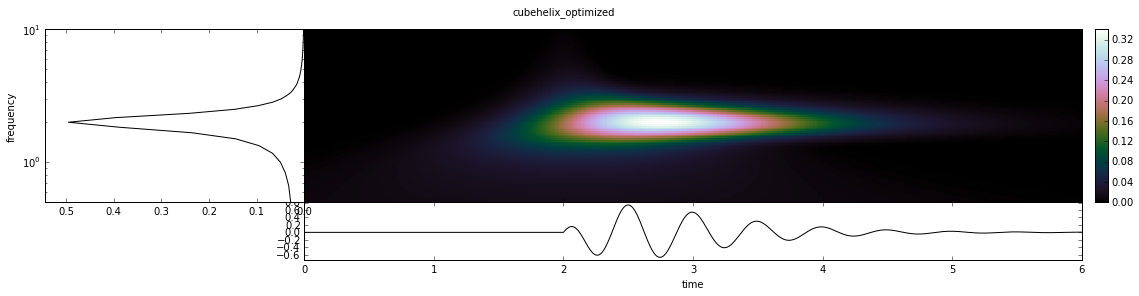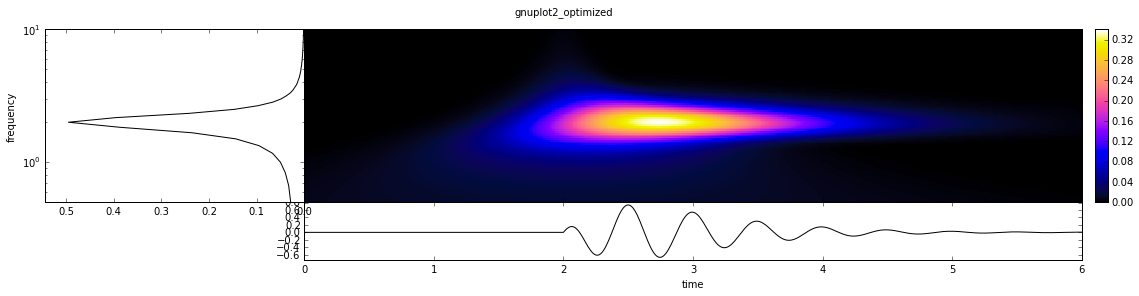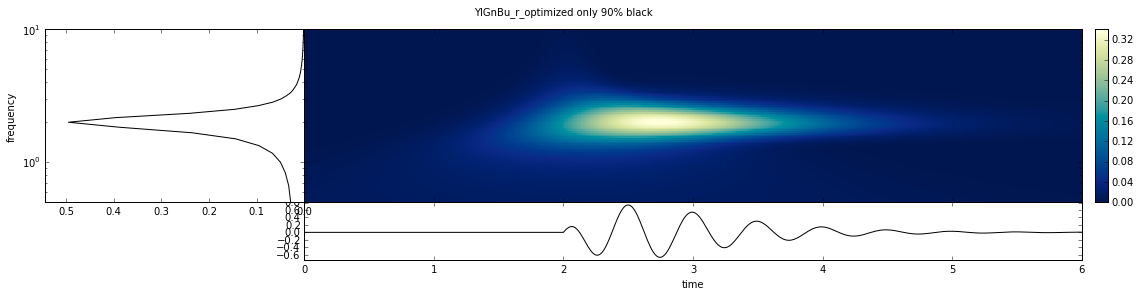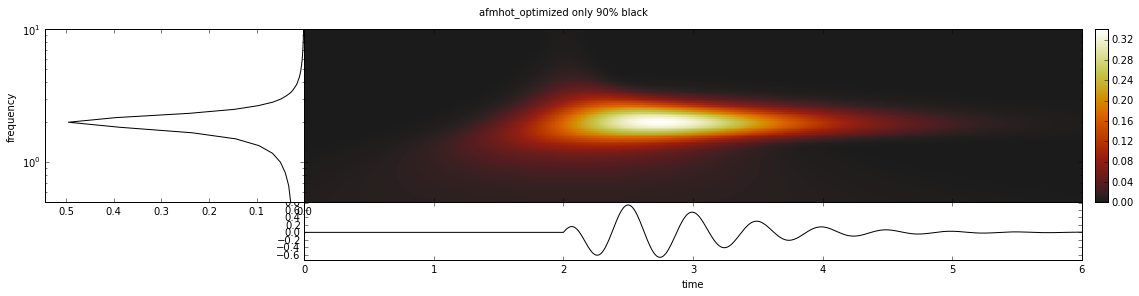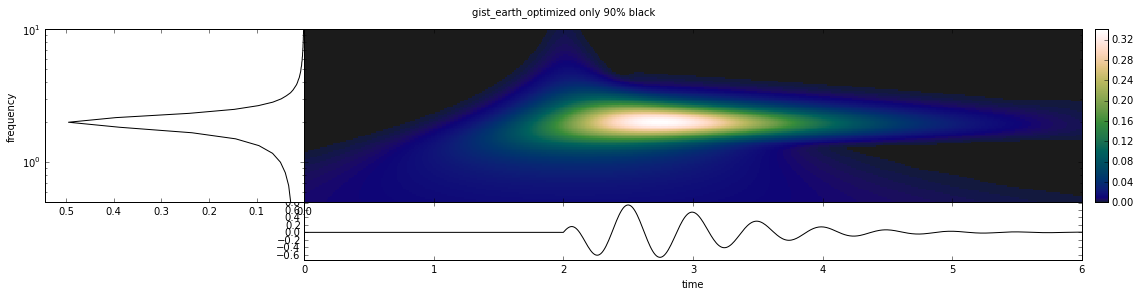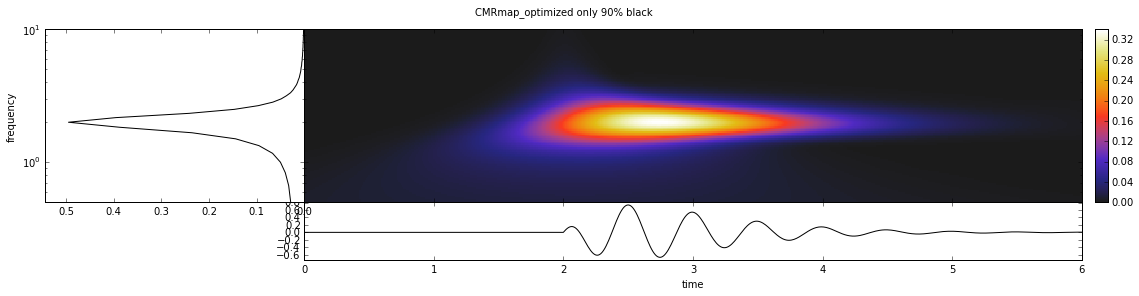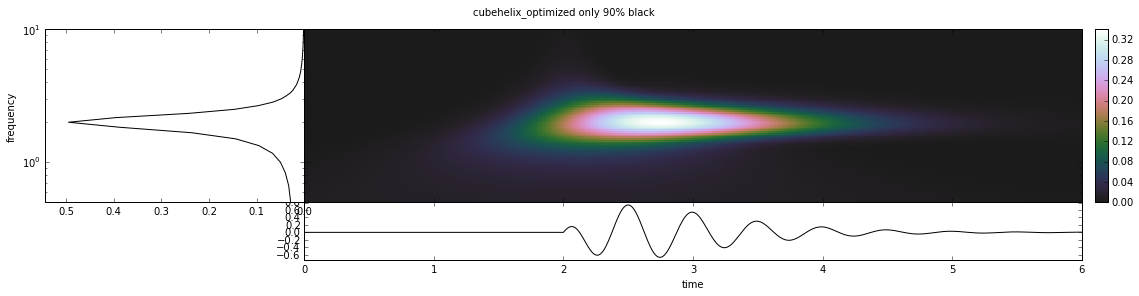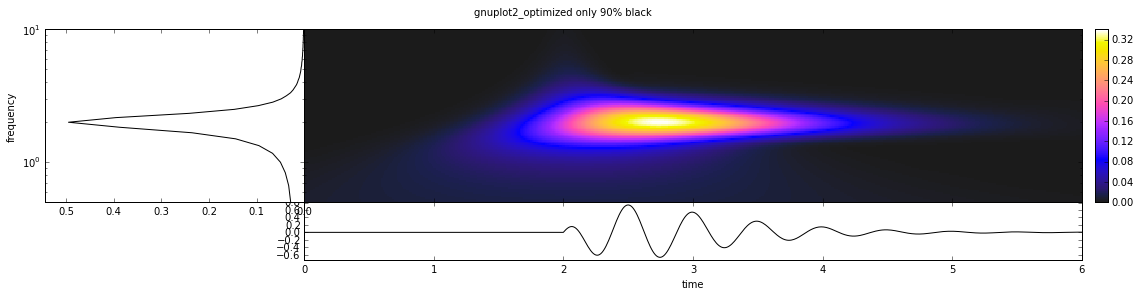# Perceptually Uniform Colormaps in Matplotlib

This notebook aims to explore and illustrate the performance of matplotlib's colormaps for qualitative plots. The most vital property of any graph is that humans are able to interpret and judge it. For plotting a collection of values this means that the perceived difference between any two values should equal the actual difference between these values. Turns out the `jet` colormap (matplotlib's default) is pretty terrible at this, as are many other colormaps.

The main reason for this is that computers usually work in the RGB color space, distances in which is are not perceptually uniform for humans. The LAB colorspace on the other hand is designed to approximate human vison. One axis is the lightness which is supposed to be linearly related to the perceived brightness. Therefore if a range of values is linearly mapped to the lightness of some colors we should be good. In this notebook we will use this as a criteria for the goodness of a colormap. Another advantage of linear lightness colormaps is that their grayscale representations are still usable which even nowadays is important for printing.

Please be aware that I have only a limited understanding of colorspaces and related concepts and don't really know what I am talking about, but there are a great number of resources out there, e.g.:

In :
```%pylab inline
from __future__ import print_function

import matplotlib
import matplotlib.pyplot as plt
import numpy as np

# Scikit-images has a bug when converting colors from LAB to RGB:
# https://github.com/scikit-image/scikit-image/issues/1201
# Thus it cannot be used here.
# import skimage.color
from colormath.color_objects import LabColor, sRGBColor
from colormath.color_conversions import convert_color
```
```Populating the interactive namespace from numpy and matplotlib
```

All colormaps shipping with matplotlib, from http://matplotlib.org/examples/color/colormaps_reference.html

Sequential ones are merged and partially reversed.

In :
```cmaps = {'sequential':     ['Blues_r', 'BuGn_r', 'BuPu_r',
'GnBu_r', 'Greens_r', 'Greys_r', 'Oranges_r', 'OrRd_r',
'PuBu_r', 'PuBuGn_r', 'PuRd_r', 'Purples_r', 'RdPu_r',
'Reds_r', 'YlGn_r', 'YlGnBu_r', 'YlOrBr_r', 'YlOrRd_r',
'afmhot', 'autumn', 'bone', 'cool', 'copper',
'gist_heat', 'gray', 'hot', 'pink',
'spring', 'summer', 'winter'],
'diverging':      ['BrBG', 'bwr', 'coolwarm', 'PiYG', 'PRGn', 'PuOr',
'RdBu', 'RdGy', 'RdYlBu', 'RdYlGn', 'Spectral',
'seismic'],
'qualitative':    ['Accent', 'Dark2', 'Paired', 'Pastel1',
'Pastel2', 'Set1', 'Set2', 'Set3'],
'misc':           ['gist_earth', 'terrain', 'ocean', 'gist_stern',
'brg', 'CMRmap', 'cubehelix',
'gnuplot', 'gnuplot2', 'gist_ncar',
'nipy_spectral', 'jet', 'rainbow',
'gist_rainbow', 'hsv', 'flag', 'prism']}
```
In :
```def optimal_lightness(npts, cmap_type, l_range=(0.0, 1.0)):
"""
Helper function defining the optimality condition for a colormap.
Depending on the colormap this might mean different things. The
l_range argument can be used to restrict the lightness range so it
does have to equal the full range.
"""
if cmap_type == "sequential_rising":
opt = np.linspace(l_range, l_range, npts)
elif cmap_type == "sequential_falling":
opt = np.linspace(l_range, l_range, npts)
elif cmap_type == "diverging_center_top":
s = npts // 2
opt = np.empty(npts)
opt[:s] = np.linspace(l_range, l_range, s)
opt[s:] = np.linspace(l_range, l_range, npts - s)
elif cmap_type == "diverging_center_bottom":
s = npts // 2
opt = np.empty(npts)
opt[:s] = np.linspace(l_range, l_range, s)
opt[s:] = np.linspace(l_range, l_range, npts - s)
elif cmap_type == "flat":
opt = np.ones(npts) * l_range
return opt
```
In :
```def plot_cmap_lightness(name, ax, cmap_type=None, l_range=(0.0, 1.0)):
"""
Plot the perceived lightness vs position in the colormap.
"""
cmap = plt.get_cmap(name)

x = np.linspace(0, 1, 256)
values = cmap(x)[:, :3]

lab_colors = []
for rgb in values:
lab_colors.append(convert_color(sRGBColor(*rgb), target_cs=LabColor))
lightness = [_i.lab_l for _i in lab_colors]

if cmap_type == "flat":
mean = np.mean(lightness) / 100.0
optimality = optimal_lightness(len(x), cmap_type=cmap_type, l_range=(mean, mean))
else:
optimality = optimal_lightness(len(x), cmap_type=cmap_type, l_range=l_range)

# Plot reference.
ax.plot(x * 100.0, optimality * 100, color="0.2", lw=0.5, ls="--")

ax.scatter(x * 100.0, lightness,
c=x, cmap=cmap, edgecolor="None",
s=60)

# Calculate "RMS Misfit" as defined by Gellar and Takeuchi 1995.
rms = np.sqrt(
np.sum((np.array(lightness) - (optimality * 100)) ** 2) / np.sum((x * 100) ** 2))

ax.plot(x * 100.0, lightness, c="0.5", lw=1)
ax.set_xlim(0, 100)
ax.set_ylim(0, 100)
ax.set_aspect("equal")
ax.text(0.02, 0.98, cmap.name, horizontalalignment='left',
verticalalignment='top', transform=ax.transAxes)
ax.text(0.98, 0.02, "RMS: %.4f" % rms, horizontalalignment='right',
verticalalignment='bottom', transform=ax.transAxes)
ax.get_xaxis().set_visible(False)
ax.get_yaxis().set_visible(False)
```

### Sequential Colormaps

Plots the lightness in the LAB color space for each colormap against the position in the colormap. The ideal curve is the dashed grey line. RMS is the root mean square misfit between the actual curve and the ideal one.

In :
```plt.figure(figsize=(18, 15))
gs = matplotlib.gridspec.GridSpec(5, 6, left=0.0, right=1.0, bottom=0.0,
top=1.0, wspace=0.0, hspace=0.0)
for g, cmap in zip(gs, cmaps["sequential"]):
plot_cmap_lightness(cmap, plt.subplot(g), cmap_type="sequential_rising")
plt.show()
```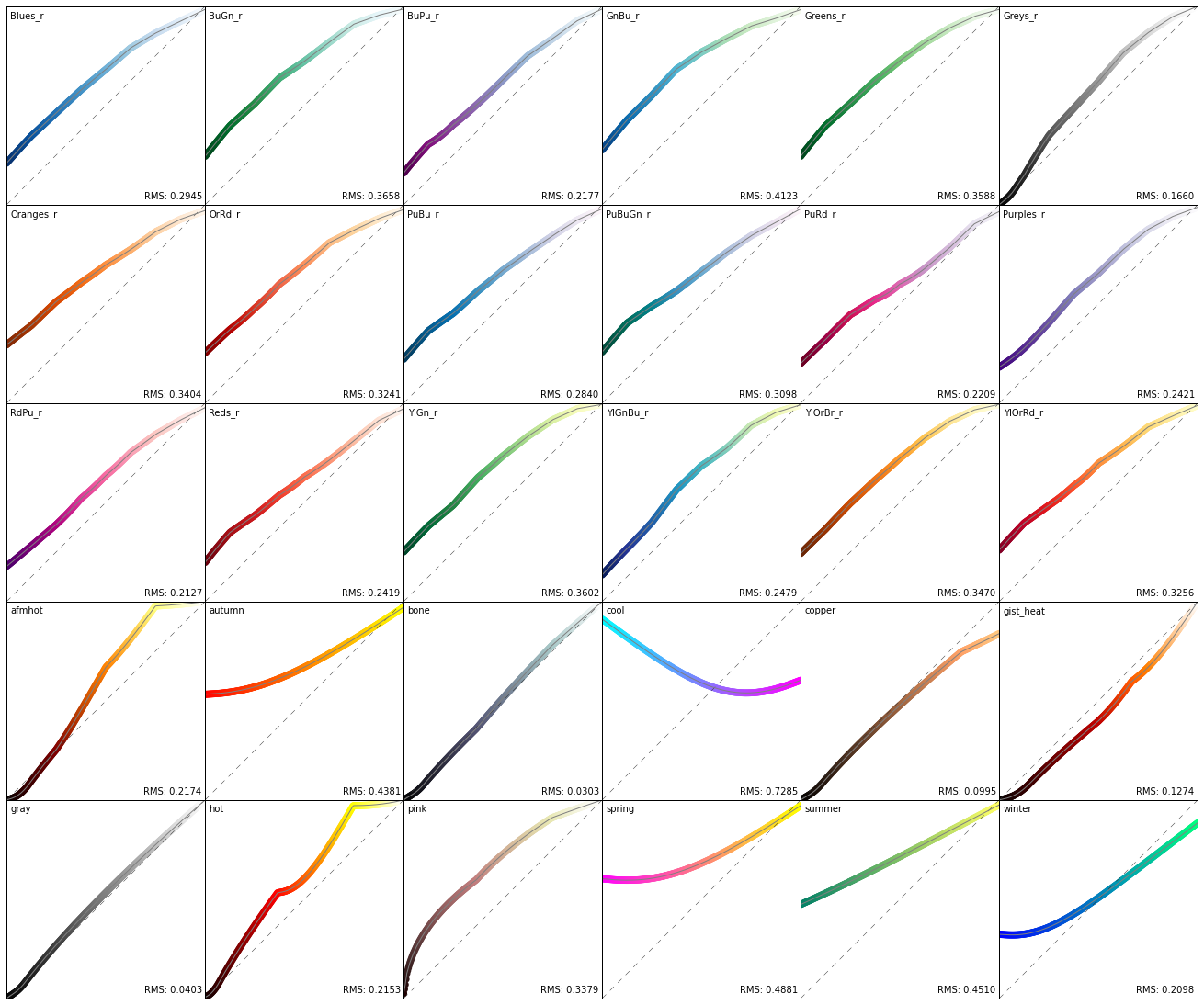### Diverging Colormaps

In :
```plt.figure(figsize=(18, 6))
gs = matplotlib.gridspec.GridSpec(2, 6, left=0.0, right=1.0, bottom=0.0,
top=1.0, wspace=0.0, hspace=0.0)
for g, cmap in zip(gs, cmaps["diverging"]):
plot_cmap_lightness(cmap, plt.subplot(g), cmap_type="diverging_center_top")
plt.show()
```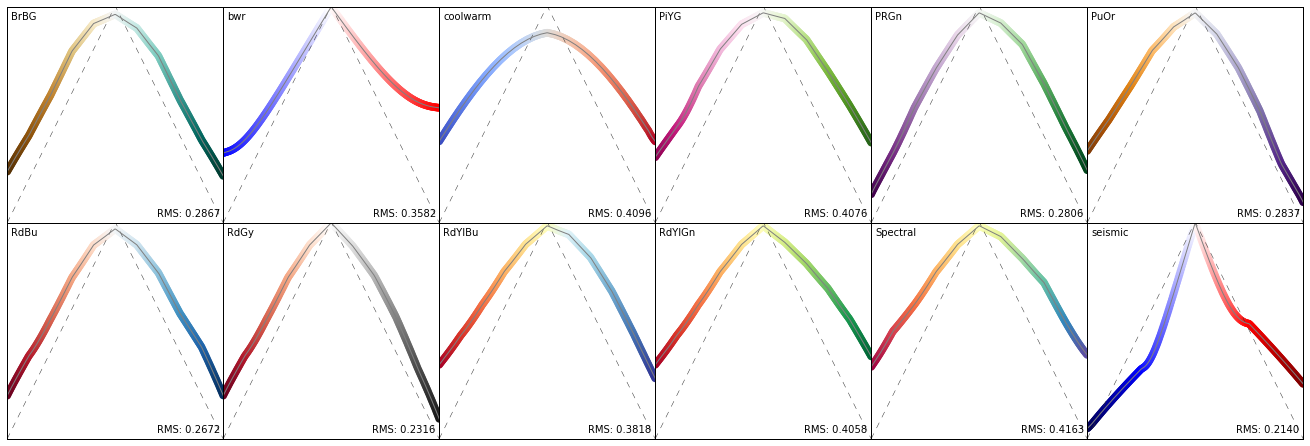### Quantitative Colormaps

In :
```plt.figure(figsize=(18, 6))
gs = matplotlib.gridspec.GridSpec(2, 6, left=0.0, right=1.0, bottom=0.0,
top=1.0, wspace=0.0, hspace=0.0)
qm = len(cmaps["qualitative"])
for g, cmap in zip([gs[_i] for _i in range(qm)], cmaps["qualitative"]):
plot_cmap_lightness(cmap, plt.subplot(g), cmap_type="flat")
plt.show()
```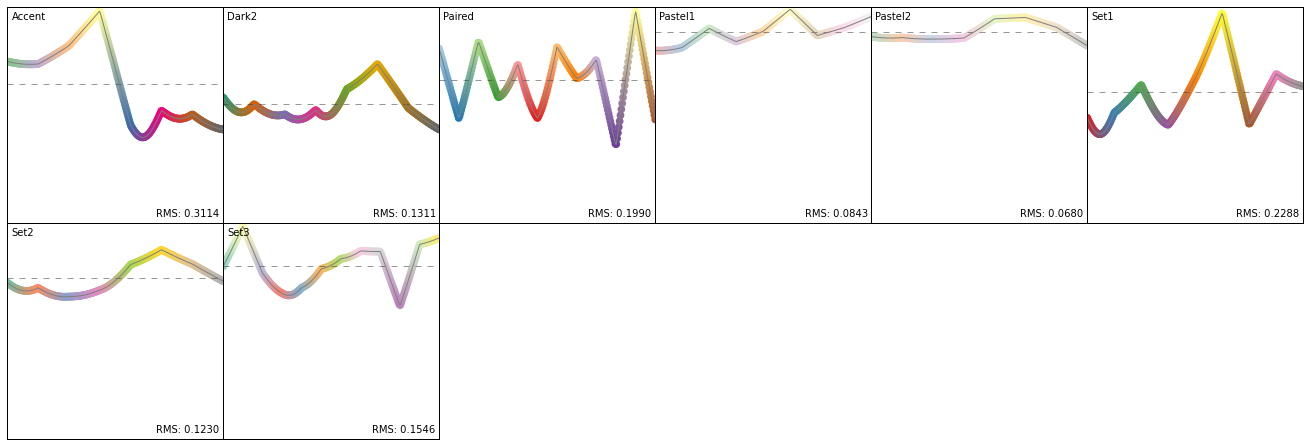### Misc Colormaps

This, amongst other things, illustrates how terrible the default `jet` colormap actually is for quantitative plots.

In :
```plt.figure(figsize=(18, 9))
gs = matplotlib.gridspec.GridSpec(3, 6, left=0.0, right=1.0, bottom=0.0,
top=1.0, wspace=0.0, hspace=0.0)
mm = len(cmaps["misc"])
for g, cmap in zip([gs[_i] for _i in range(mm)], cmaps["misc"]):
plot_cmap_lightness(cmap, plt.subplot(g), cmap_type="sequential_rising")
plt.show()
```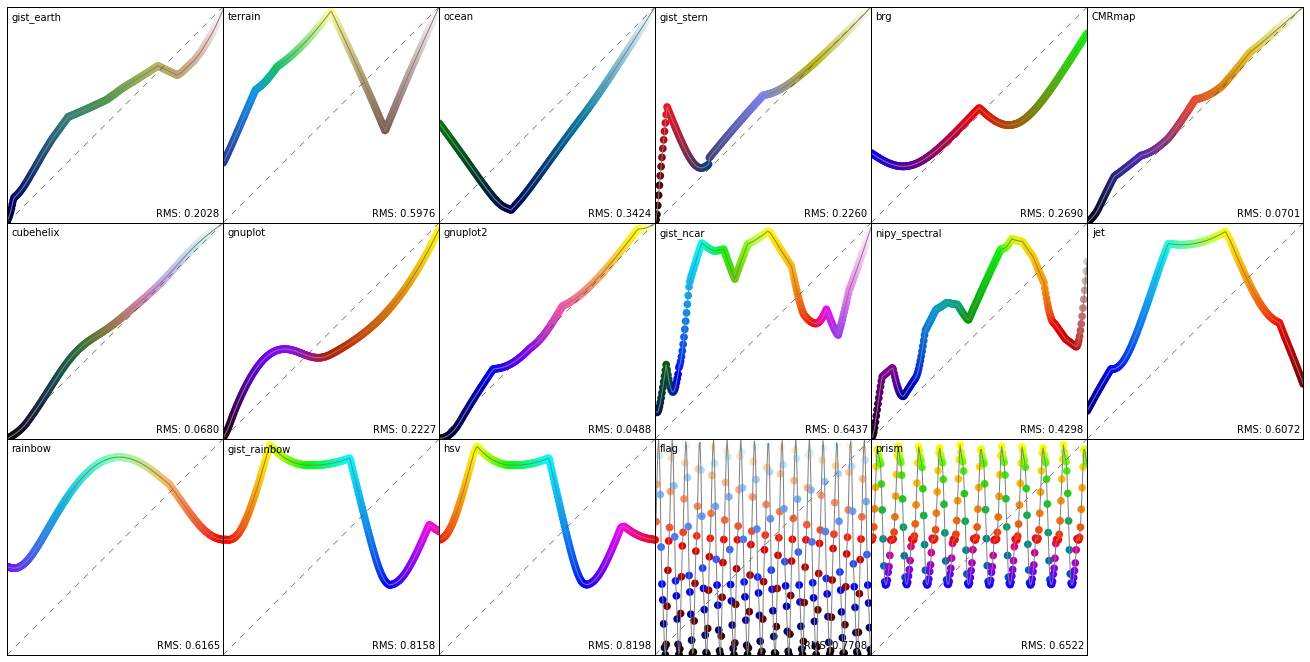## "Optimal" Colormaps

The idea of modifying each colormap to achieve "optimal" lightness immediatly comes to mind. This is really easy to implement by just going to the LAB color space, setting the lightness to the desired value, and going back to RGB. We already got the functionality to acquire the target lightness (the `optimal_lightness()` fct) so this is straightforward to do.

A complication arises as the LAB color space is larger then the sRGB one. Therefore some values must be clamped when going back to RGB. This is also the reason for the bumps in quite some of the resulting colormaps. To get rid of these bumps I guess one could use some sampling strategy like simulated annealing while using similarity to the original colormap as an additional criteria for the cost function. People that actually know something about colorspaces might also be able to come up with an analytic solution...

Nonetheless, the results are quite pleasing in my opinion. I think the results should look quite good on a PC with all the colorspace conversions between programs, OS, and the final output. To have an optimal printed output the whole thing should probably be done in the CMYK colorspace or whatever else printers use.

In :
```def optimize_colormap(name, cmap_type=None, l_range=(0.0, 1.0)):
cmap = plt.get_cmap(name)
x = np.linspace(0, 1, 256)
values = cmap(x)[:, :3]
lab_colors = []
for rgb in values:
lab_colors.append(convert_color(sRGBColor(*rgb), target_cs=LabColor))

if cmap_type == "flat":
mean = np.mean([_i.lab_l for _i in lab_colors])
target_lightness = optimal_lightness(len(x), cmap_type=cmap_type, l_range=(mean, mean))
else:
target_lightness = optimal_lightness(len(x), cmap_type=cmap_type, l_range=l_range) * 100.0

for color, lightness in zip(lab_colors, target_lightness):
color.lab_l = lightness
# Go back to rbg.
rgb_colors = [convert_color(_i, target_cs=sRGBColor) for _i in lab_colors]
# Clamp values as colorspace of LAB is larger then sRGB.
rgb_colors = [(_i.clamped_rgb_r, _i.clamped_rgb_g, _i.clamped_rgb_b) for _i in rgb_colors]

cm = matplotlib.colors.LinearSegmentedColormap.from_list(name=name + "_optimized", colors=rgb_colors)
return cm
```

### "Optimal" Sequential Colormaps

In :
```plt.figure(figsize=(18, 15))
gs = matplotlib.gridspec.GridSpec(5, 6, left=0.0, right=1.0, bottom=0.0,
top=1.0, wspace=0.0, hspace=0.0)
for g, cmap in zip(gs, cmaps["sequential"]):
cm = optimize_colormap(cmap, cmap_type="sequential_rising")
plot_cmap_lightness(cm, plt.subplot(g), cmap_type="sequential_rising")
plt.show()
```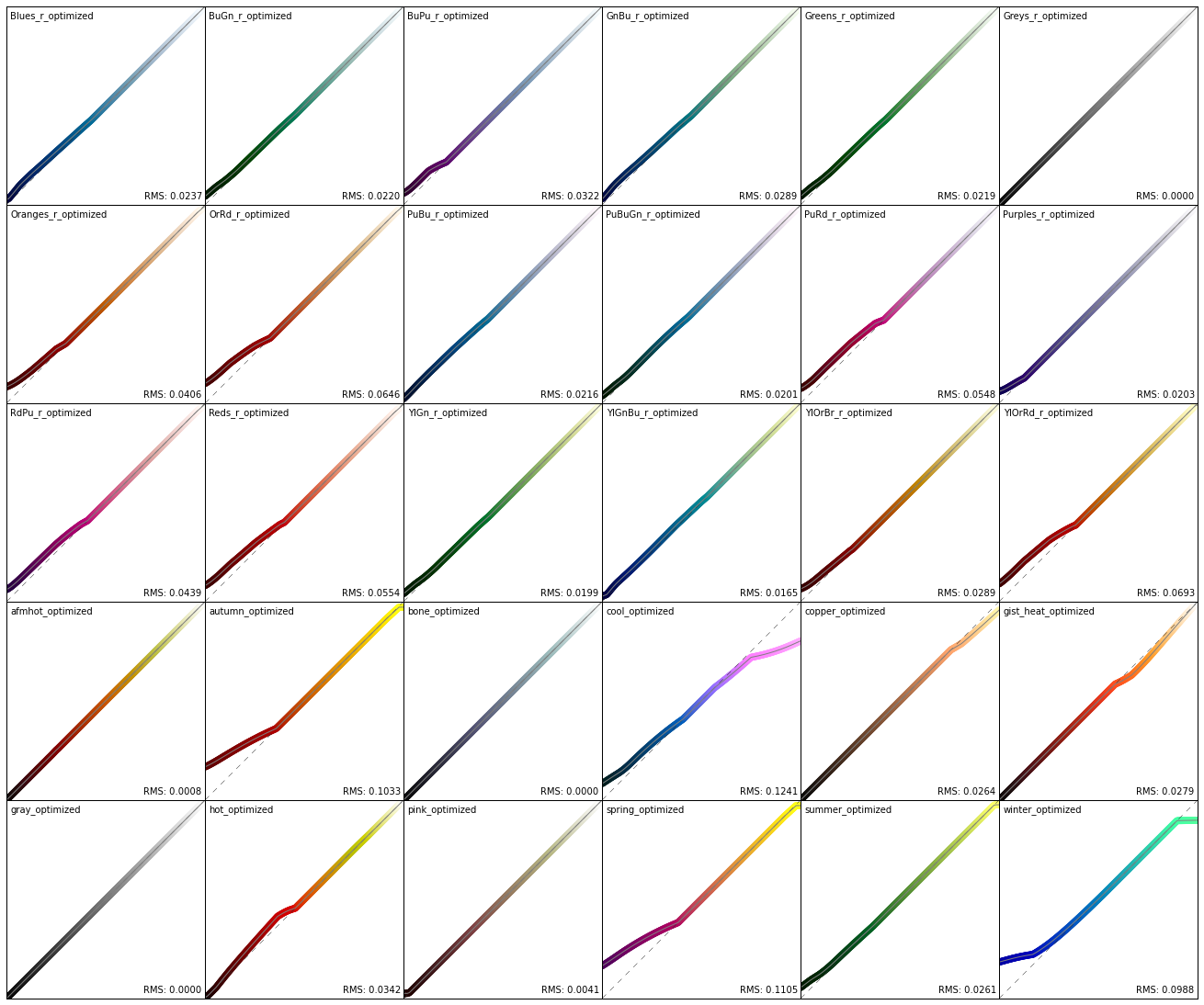### "Optimal" Diverging Colormaps

In :
```plt.figure(figsize=(18, 6))
gs = matplotlib.gridspec.GridSpec(2, 6, left=0.0, right=1.0, bottom=0.0,
top=1.0, wspace=0.0, hspace=0.0)
for g, cmap in zip(gs, cmaps["diverging"]):
cm = optimize_colormap(cmap, cmap_type="diverging_center_top")
plot_cmap_lightness(cm, plt.subplot(g), cmap_type="diverging_center_top")
plt.show()
```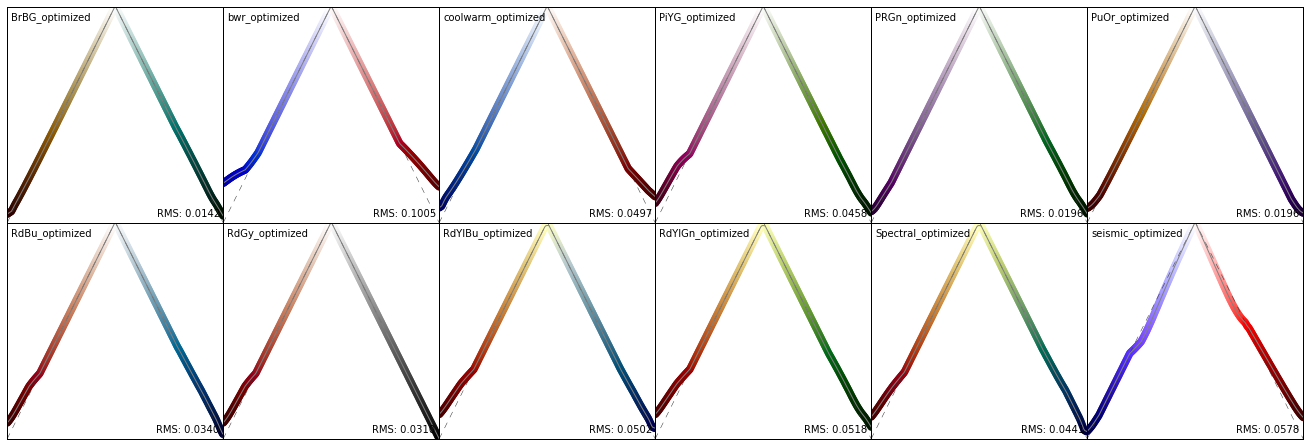### "Optimal" Qualitative Colormaps

An optimal qualitative colormap should have uniform lightness for all of its values to not prefer certain values over others. Here the target lightness is the mean lightness before the optimization.

In :
```plt.figure(figsize=(18, 6))
gs = matplotlib.gridspec.GridSpec(2, 6, left=0.0, right=1.0, bottom=0.0,
top=1.0, wspace=0.0, hspace=0.0)
qm = len(cmaps["qualitative"])
for g, cmap in zip([gs[_i] for _i in range(qm)], cmaps["qualitative"]):
cm = optimize_colormap(cmap, cmap_type="flat")
plot_cmap_lightness(cm, plt.subplot(g), cmap_type="flat")
plt.show()
```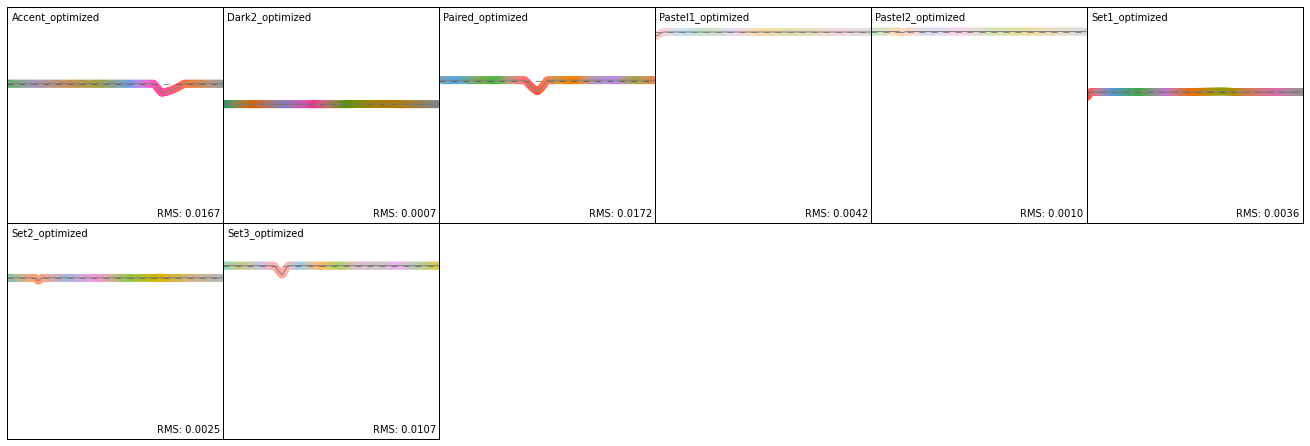### "Optimal" Misc Colormaps

For some colormaps this is a bit pointless but many actually make pretty good sequential colormaps. Even `jet` becomes a fairly reasonable choice.

In :
```plt.figure(figsize=(18, 9))
gs = matplotlib.gridspec.GridSpec(3, 6, left=0.0, right=1.0, bottom=0.0,
top=1.0, wspace=0.0, hspace=0.0)
mm = len(cmaps["misc"])
for g, cmap in zip([gs[_i] for _i in range(mm)], cmaps["misc"]):
cm = optimize_colormap(cmap, cmap_type="sequential_rising")
plot_cmap_lightness(cm, plt.subplot(g), cmap_type="sequential_rising")
plt.show()
```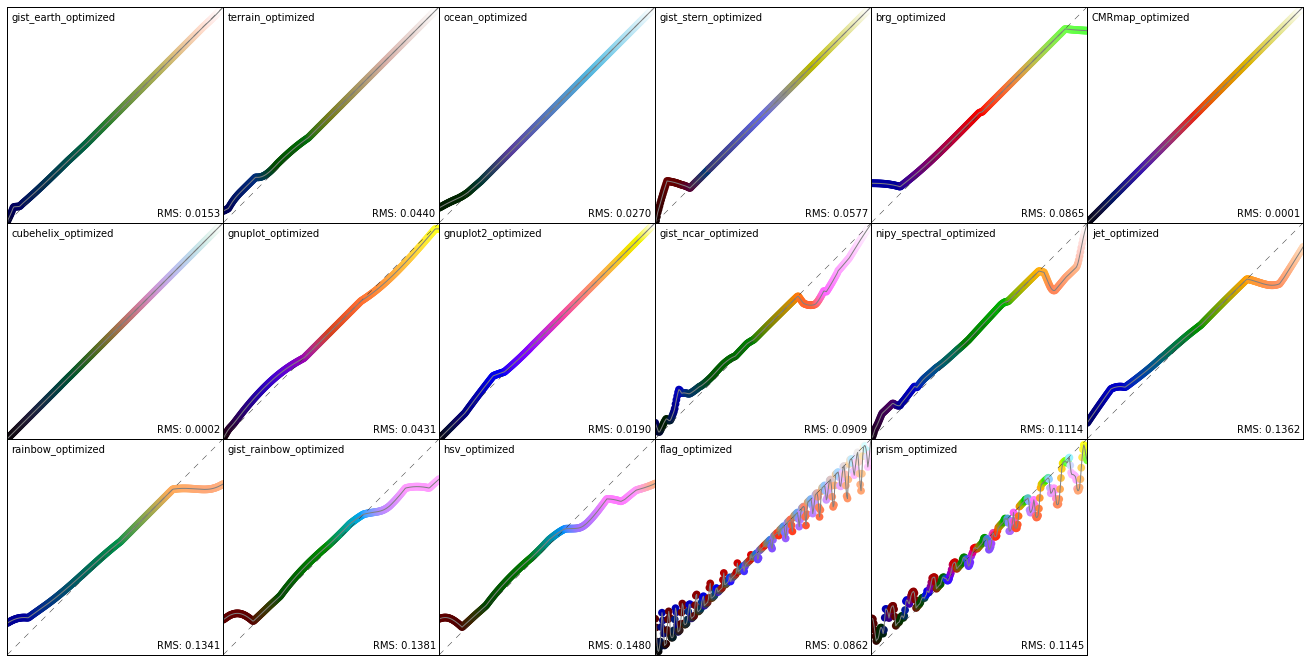## Good Default Sequential Colormap

The following are six hand picked examples to test in a real world case. They are tested twice, once with full range lightness, once where the bottom 10% are not used to potentially get an asthetically more pleasing result.

In :
```chosen_cmaps = ["YlGnBu_r", "afmhot", "gist_earth", "CMRmap", "cubehelix", "gnuplot2"]

full_range_cmaps = [optimize_colormap(_i, cmap_type="sequential_rising") for _i in chosen_cmaps]
# All black might not look asthetically pleasing.
ninety_percent_cmaps = [optimize_colormap(_i, cmap_type="sequential_rising", l_range=(0.1, 1.0)) for _i in chosen_cmaps]

print("Full range lightness")
plt.figure(figsize=(18, 3))
gs = matplotlib.gridspec.GridSpec(1, 6, left=0.0, right=1.0, bottom=0.0,
top=1.0, wspace=0.0, hspace=0.0)
for g, cmap in zip(gs, full_range_cmaps):
plot_cmap_lightness(cmap, plt.subplot(g), cmap_type="sequential_rising")
plt.show()

print("Only 90% black")
plt.figure(figsize=(18, 3))
gs = matplotlib.gridspec.GridSpec(1, 6, left=0.0, right=1.0, bottom=0.0,
top=1.0, wspace=0.0, hspace=0.0)
for g, cmap in zip(gs, ninety_percent_cmaps):
plot_cmap_lightness(cmap, plt.subplot(g), cmap_type="sequential_rising", l_range=(0.1, 1.0))
plt.show()
```
```Full range lightness
```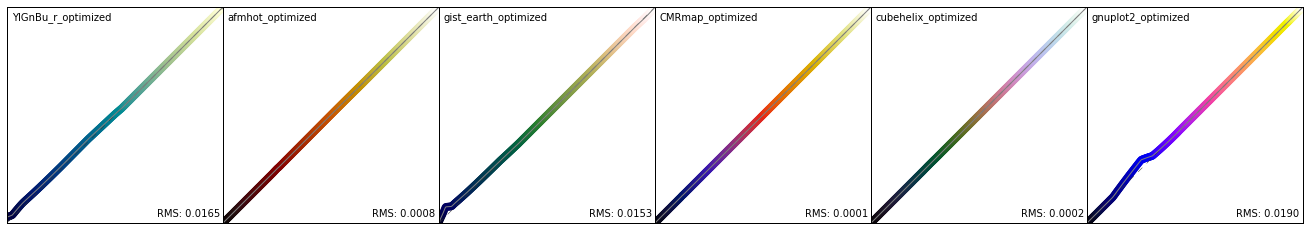```Only 90% black
```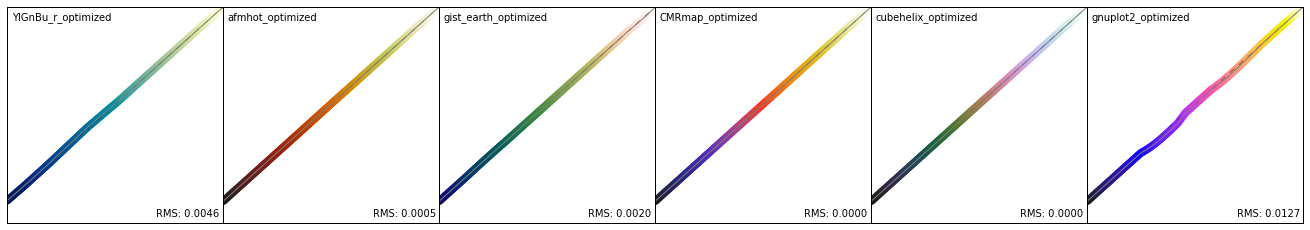### Spectrogram Example

In :
```from obspy import read

plt.close()
st.spectrogram(log=True, title="Current Default", show=False)
plt.gcf().set_size_inches(18, 4)
plt.show()

for cmap in full_range_cmaps:
plt.close()
st.spectrogram(log=True, title=cmap.name, cmap=cmap, show=False)
plt.gcf().set_size_inches(18, 4)
plt.show()

for cmap in ninety_percent_cmaps:
plt.close()
st.spectrogram(log=True, title=cmap.name + " only 90% black",
cmap=cmap, show=False)
plt.gcf().set_size_inches(18, 4)
plt.show()
```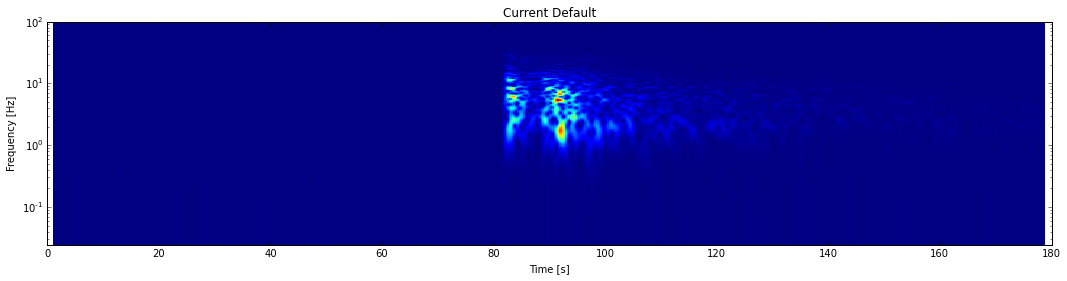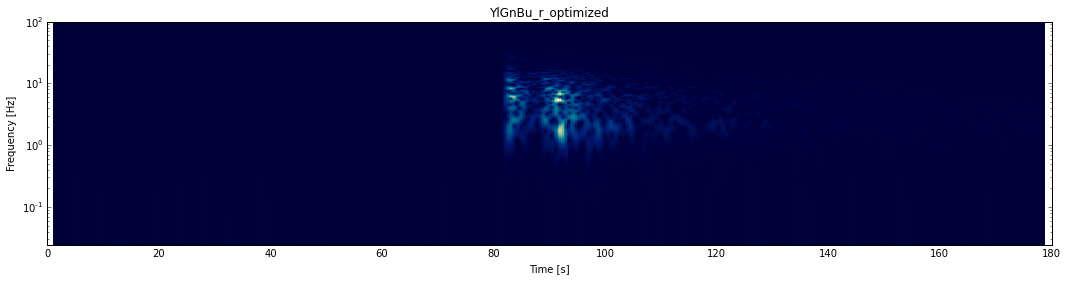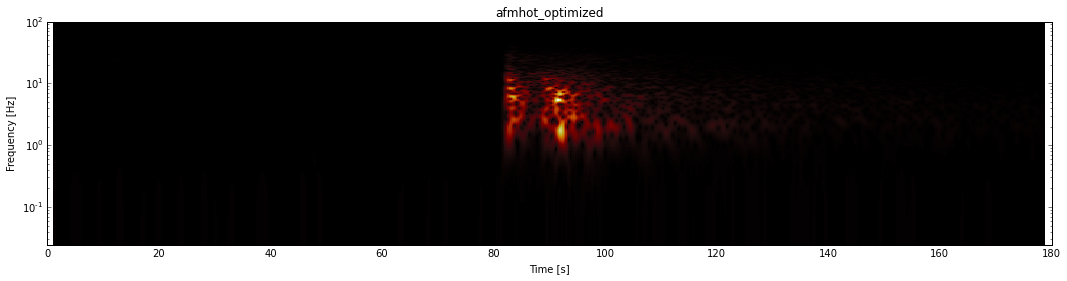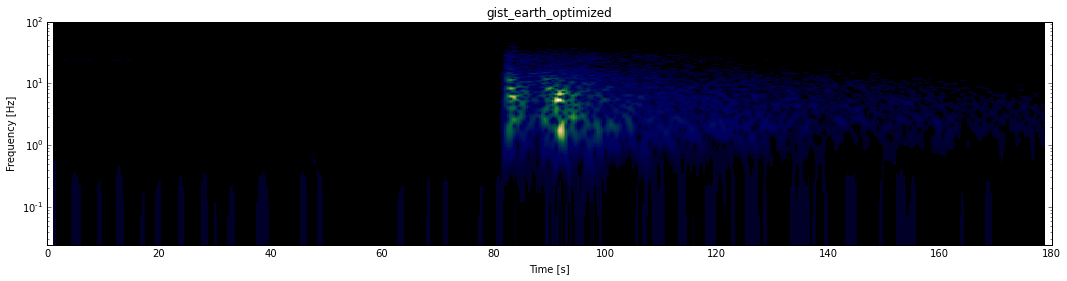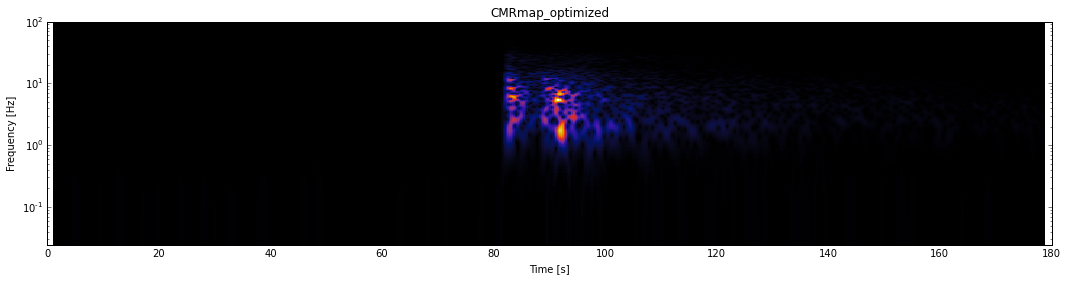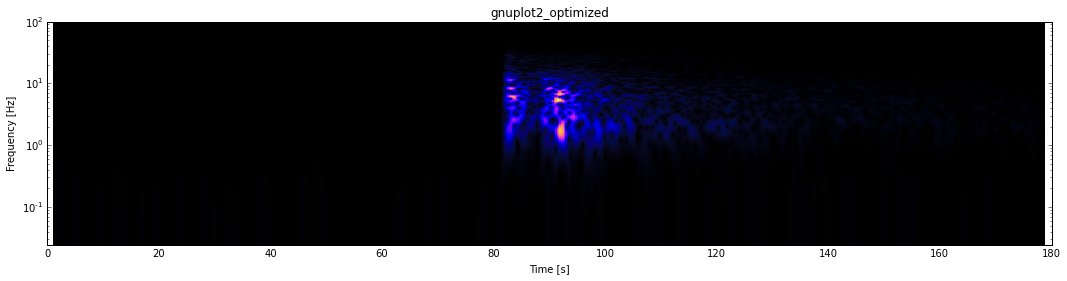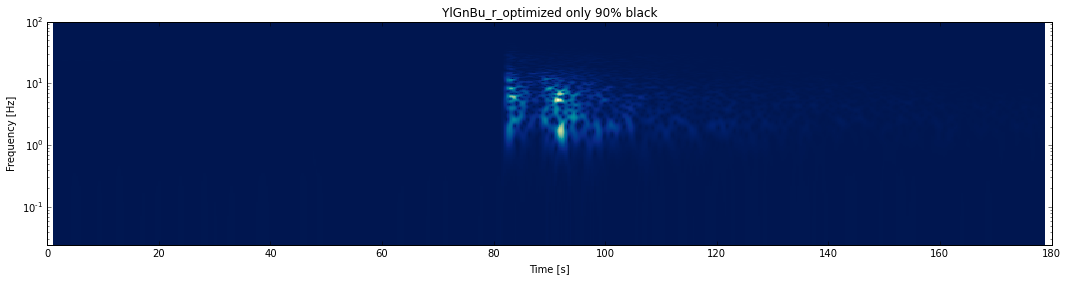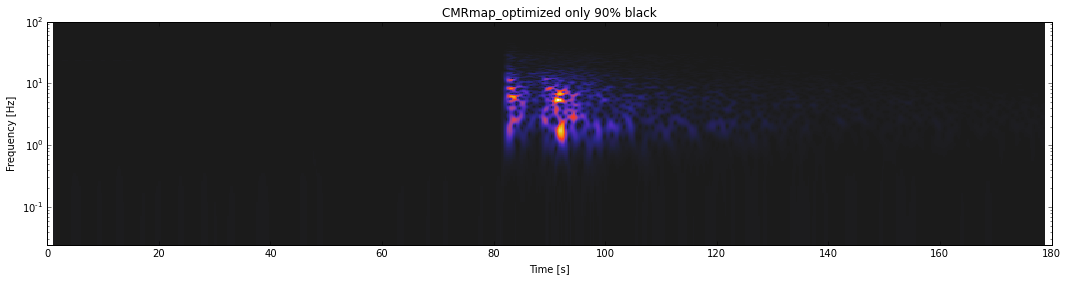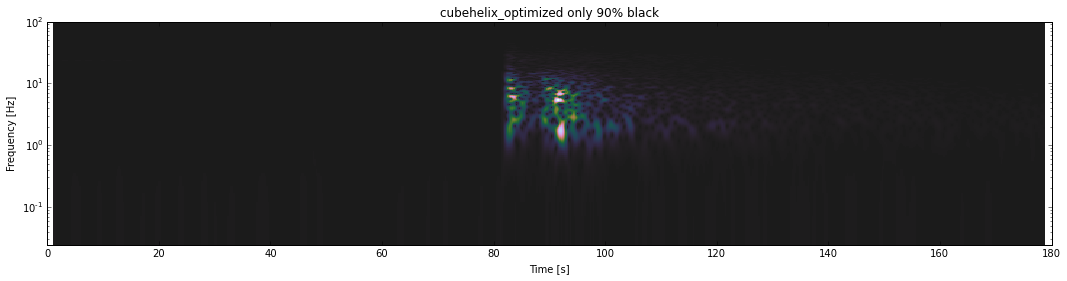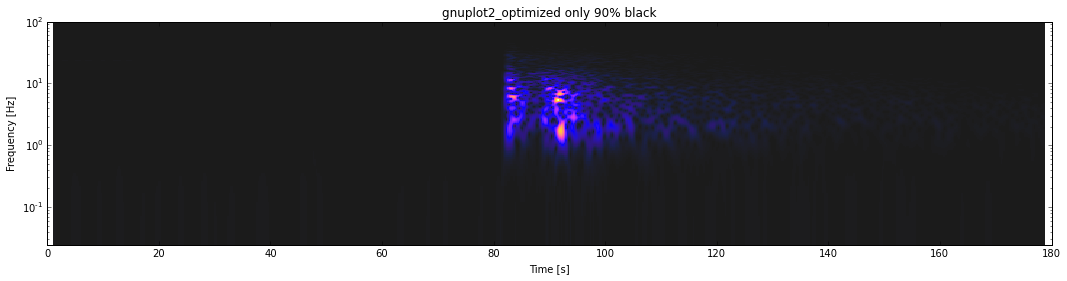### Time Frequency Misfit Example

In :
```from obspy.signal.tf_misfit import plotTfr

# general constants
tmax = 6.
dt = 0.01
npts = int(tmax / dt + 1)
t = np.linspace(0., tmax, npts)

fmin = .5
fmax = 10

# constants for the signal
A1 = 4.
t1 = 2.
f1 = 2.
phi1 = 0.

# generate the signal
H1 = (np.sign(t - t1) + 1) / 2
st1 = A1 * (t - t1) * np.exp(-2 * (t - t1)) * \
np.cos(2. * np.pi * f1 * (t - t1) + phi1 * np.pi) * H1

plotTfr(st1, dt=dt, fmin=fmin, fmax=fmax, show=False)
plt.gcf().set_size_inches(18, 4)
plt.suptitle("Current Default")
plt.show()

for cmap in full_range_cmaps:
plotTfr(st1, dt=dt, fmin=fmin, fmax=fmax, show=False, cmap=cmap)
plt.gcf().set_size_inches(18, 4)
plt.suptitle(cmap.name)
plt.show()

for cmap in ninety_percent_cmaps:
plotTfr(st1, dt=dt, fmin=fmin, fmax=fmax, show=False, cmap=cmap)
plt.gcf().set_size_inches(18, 4)
plt.suptitle(cmap.name + " only 90% black")
plt.show()
```